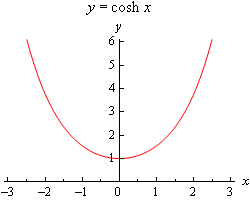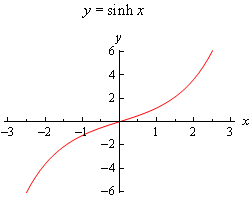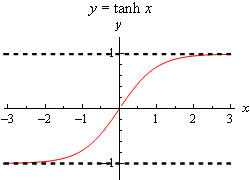Paul's Online Notes
Home / Calculus I / Derivatives / Derivatives of Hyperbolic Functions
Show Mobile Notice Show All Notes Hide All Notes
Mobile Notice
You appear to be on a device with a "narrow" screen width (i.e. you are probably on a mobile phone). Due to the nature of the mathematics on this site it is best views in landscape mode. If your device is not in landscape mode many of the equations will run off the side of your device (should be able to scroll to see them) and some of the menu items will be cut off due to the narrow screen width.

### Section 3.8 : Derivatives of Hyperbolic Functions

The last set of functions that we’re going to be looking in this chapter at are the hyperbolic functions. In many physical situations combinations of $${{\bf{e}}^x}$$ and $${{\bf{e}}^{ - x}}$$ arise fairly often. Because of this these combinations are given names. There are six hyperbolic functions and they are defined as follows.

$\begin{array}{ll}\sinh x = \displaystyle \frac{{{{\bf{e}}^x} - {{\bf{e}}^{ - x}}}}{2} & \hspace{1.0in}\cosh x = \displaystyle \frac{{{{\bf{e}}^x} + {{\bf{e}}^{ - x}}}}{2}\\ \tanh x = \displaystyle \frac{{\sinh x}}{{\cosh x}} & \hspace{1.0in}\coth x = \displaystyle \frac{{\cosh x}}{{\sinh x}} = \displaystyle \frac{1}{{\tanh x}}\\ {\mbox{sech}}\,x = \displaystyle \frac{1}{{\cosh x}} & \hspace{1.0in}{\mbox{csch}}\,x = \displaystyle \frac{1}{{\sinh x}}\end{array}$

Here are the graphs of the three main hyperbolic functions.We also have the following facts about the hyperbolic functions.

$\begin{array}{ll}\sinh \left( { - x} \right) = - \sinh \left( x \right) & \hspace{1.0in}\cosh \left( { - x} \right) = \cosh \left( x \right)\\ {\cosh ^2}\left( x \right) - {\sinh ^2}\left( x \right) = 1 & \hspace{1.0in}1 - {\tanh ^2}\left( x \right) = {\mbox{sec}}{{\mbox{h}}^{\mbox{2}}}\left( x \right)\end{array}$

You’ll note that these are similar, but not quite the same, to some of the more common trig identities so be careful to not confuse the identities here with those of the standard trig functions.

Because the hyperbolic functions are defined in terms of exponential functions finding their derivatives is fairly simple provided you’ve already read through the next section. We haven’t however so we’ll need the following formula that can be easily proved after we’ve covered the next section.

$\frac{d}{{dx}}\left( {{{\bf{e}}^{ - x}}} \right) = - {{\bf{e}}^{ - x}}$

With this formula we’ll do the derivative for hyperbolic sine and leave the rest to you as an exercise.

$\frac{d}{{dx}}\left( {\sinh x} \right) = \frac{d}{{dx}}\left( {\frac{{{{\bf{e}}^x} - {{\bf{e}}^{ - x}}}}{2}} \right) = \frac{{{{\bf{e}}^x} - \left( { - {{\bf{e}}^{ - x}}} \right)}}{2} = \frac{{{{\bf{e}}^x} + {{\bf{e}}^{ - x}}}}{2} = \cosh x$

For the rest we can either use the definition of the hyperbolic function and/or the quotient rule. Here are all six derivatives.

$\begin{array}{ll}\displaystyle \frac{d}{{dx}}\left( {\sinh x} \right) = \cosh x & \hspace{1.0in} \displaystyle \frac{d}{{dx}}\left( {\cosh x} \right) = \sinh x\\ \displaystyle \frac{d}{{dx}}\left( {\tanh x} \right) = \mbox{sech}^{2}x & \hspace{1.0in} \displaystyle \frac{d}{{dx}}\left( {\coth x} \right) = - {\mbox{csch}}^{2}x\\ \displaystyle \frac{d}{{dx}}\left( {{\mbox{sech}}\,x} \right) = - {\mbox{sech }}x{\mbox{ tanh }}x & \hspace{1.0in} \displaystyle \frac{d}{{dx}}\left( {{\mbox{csch}}\,x} \right) = - {\mbox{csch }}x{\mbox{ coth }}x\end{array}$

Here are a couple of quick derivatives using hyperbolic functions.

Example 1 Differentiate each of the following functions.
1. $$f\left( x \right) = 2{x^5}\cosh x$$
2. $$\displaystyle h\left( t \right) = \frac{{\sinh t}}{{t + 1}}$$
Show Solution

a

$f'\left( x \right) = 10{x^4}\cosh x + 2{x^5}\sinh x$

b

$h'\left( t \right) = \frac{{\left( {t + 1} \right)\cosh t - \sinh t}}{{{{\left( {t + 1} \right)}^2}}}$